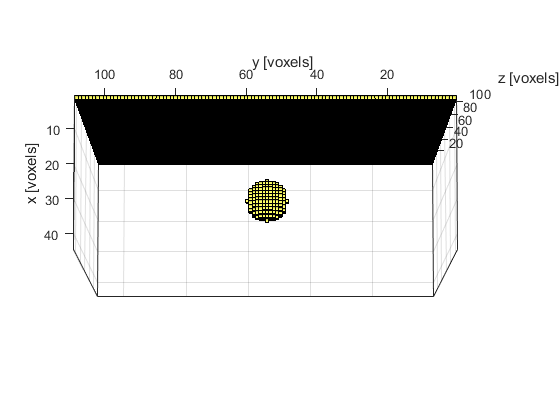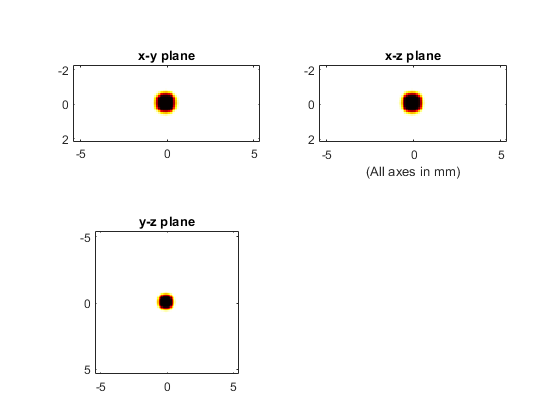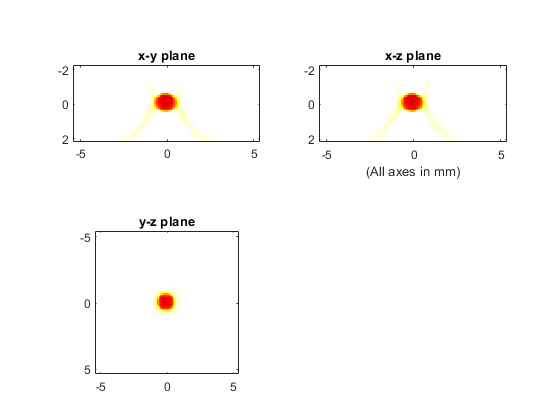# 3D FFT Reconstruction For A Planar Sensor Example

This example demonstrates the use of k-Wave for the reconstruction of a three-dimensional photoacoustic wave-field recorded over a planar array of sensor elements. The sensor data is simulated using `kspaceFirstOrder3D` and reconstructed using `kspacePlaneRecon`. It builds on the Simulations In Three Dimensions and 2D FFT Reconstruction For A Line Sensor examples.

## Simulating the sensor data

The sensor data is simulated using `kspaceFirstOrder3D` in the same way as in the preceding simulation examples. The initial pressure is created using `makeBall` and is again smoothed prior to invoking the simulation. The sensor mask is defined to be a planar sensor array (over a plane x = const). A visualisation of the initial pressure distribution and the sensor mask using `voxelPlot` is given below.

```% smooth the initial pressure distribution and restore the magnitude
source.p0 = smooth(p0, true);

% define a binary planar sensor
```## Performing the reconstruction

The reconstruction is invoked by calling `kspacePlaneRecon` with the sensor data (recorded over a planar sensor array), as well as the properties of the acoustic medium and the sampling parameters. By default, the sensor data input must be indexed as `p_tyz(time, sensor_y_position, sensor_z_position)`. Alternatively, the optional input parameter `'DataOrder'` can be set to `'yzt'` (the default setting is `'tyz'`). In both cases, the simulated `sensor_data` returned by `kspaceFirstOrder3D` must first be reshaped.

```% reshape sensor data to y, z, t
sensor_data_rs = reshape(sensor_data, Ny, Nz, kgrid.Nt);

% reconstruct the initial pressure
p_xyz = kspacePlaneRecon(sensor_data_rs, kgrid.dy, kgrid.dz, kgrid.dt, ...
medium.sound_speed, 'DataOrder', 'yzt', 'PosCond', true, 'Plot', true);
```

The size of the recorded data and the time to compute the reconstruction are both printed to the command line.

```Running k-Wave planar reconstruction...
grid size: 212 by 44 by 44 grid points
interpolation mode: *nearest
applying positivity condition...
computation completed in 0.14516s
```

A plot of the initial pressure distribution and the reconstructed initial pressure distribution with a positivity condition (interpolated onto a k-space grid with the same dimensions) are shown below. The three displayed image planes intersect at the centre of ball shown above. An animated slice-by-slice visualisation of the reconstructed initial pressure distribution can also be viewed using `flyThrough`.